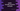# Find the maximum of two or three values in Kotlin using maxOf function# Kotlin maxOf function example to find the maximum :

Kotlin standard library comes with a lot of different functions derived under different packages. For example, if we want to find out the largest of two or three numbers in Kotlin, we can either write our own comparison function or use the one that is provided under the standard library.

In this tutorial, we will learn how to compare two or three values quickly in Kotlin using its predefined standard library function maxOf. We will learn different variants of this function with examples.

maxOf _is defined in ‘kotlin.comparisons’_ package.

## maxOf function to compare two arguments :

Let’s try to compare two arguments first. The following function is used to find out the greater argument between two :

``fun maxOf(a: T, b: T): T``

It returns the greater of the two values passing as arguments. If both values are equal, it will return the first one. Kotlin also provides separate functions for Byte, Short, Int, Long, Float, and _Double _values comparison. Following are these functions :

``````fun maxOf(a: Byte, b: Byte): Byte
fun maxOf(a: Short, b: Short): Short
fun maxOf(a: Int, b: Int): Int
fun maxOf(a: Long, b: Long): Long
fun maxOf(a: Float, b: Float): Float
fun maxOf(a: Double, b: Double): Double``````

Same as the above, it returns the maximum value of two passed arguments.

We have one more variant of _maxOf _:

``fun  maxOf(a: T, b: T, comparator: Comparator): T``

It takes one more third variable, a Comparator. The values are compared using the Comparator _and the _maximum value is returned. If both are the same, the first one is returned.

## Example :

Let’s try to understand the above function with an example :

``````data class Student(val age: Int) : Comparable {
override fun compareTo(other: Student) = other.age - age
}

fun main(args: Array) {
//1
println("Byte comparision \${maxOf(1.toByte(), 2.toByte())}")
println("Short comparision \${maxOf(1.toShort(), 2.toShort())}")
println("Integer comparision \${maxOf(1, 2)}")
println("Long comparision \${maxOf(1L, 2L)}")
println("Float comparision \${maxOf(1.2f, 2.4f)}")
println("Double comparision \${maxOf(1.34, 2.44)}")

//2
val student1 = Student(12)
val student2 = Student(22)

//3
println(maxOf(student1, student2))
println(maxOf(student1, student2, compareBy { it.age }))
}``````
``````Byte comparision 2
Short comparision 2
Integer comparision 2
Long comparision 2
Float comparision 2.4
Double comparision 2.44
Student(age=12)
Student(age=22)``````

### Explanation :

The commented numbers in the above program denote the step numbers below :

1. The first 6 println statements are for showing the comparison between Byte, Short, Int, Long, Float, and Double values. As you can see that all statements are printing out the larger value each time.
2. To compare two custom objects, we have created one data class Student implementing the interface Comparable. The only property of this class is age. We have created two Student variables student1 and student2 with age 12 and 22.
3. The first statement is printing the larger Student variable by comparing it using its own Comparable. But the second statement is comparing the variables by using the new Comparable. The first print statement printed the object with minimum age and the second statement printed the object with maximum age.

## maxOf function to compare three arguments :

maxOf _is also available for comparing _three arguments. The functions are similar for three arguments as well. Following are the available functions :

``````fun maxOf(a: Byte, b: Byte, c: Byte): Byte
fun maxOf(a: Short, b: Short, c: Short): Short
fun maxOf(a: Int, b: Int, c: Int): Int
fun maxOf(a: Long, b: Long, c: Long): Long
fun maxOf(a: Float, b: Float, c: Float): Float
fun maxOf(a: Double, b: Double, c: Double): Double``````

With _Comparable _:

``````fun  maxOf(a: T, b: T, c: T): T
fun  maxOf(a: T, b: T, c: T,comparator: Comparator): T``````

### Example :

``````data class Student(val age: Int) : Comparable {
override fun compareTo(other: Student) = other.age - age
}

fun main(args: Array) {
println("Byte comparision \${maxOf(1.toByte(), 2.toByte(), 3.toByte())}")
println("Short comparision \${maxOf(1.toShort(), 2.toShort(), 3.toShort())}")
println("Integer comparision \${maxOf(1, 2, 3)}")
println("Long comparision \${maxOf(1L, 2L, 3L)}")
println("Float comparision \${maxOf(1.2f, 2.4f, 3.4f)}")
println("Double comparision \${maxOf(1.34, 2.44, 3.65)}")

val student1 = Student(12)
val student2 = Student(22)
val student3 = Student(32)

println(maxOf(student1, student2, student3))
println(maxOf(student1, student2, student3, compareBy { it.age }))
}``````
``````Byte comparision 3
Short comparision 3
Integer comparision 3
Long comparision 3
Float comparision 3.4
Double comparision 3.65
Student(age=12)
Student(age=32)``````

As you can see that these examples are the same as the previous one we have explained with two arguments.

These examples are also available on Github.

### Conclusion :

maxOf _function comes in handy for quickly comparing _two or three values in Kotlin. We can even use this method for comparing custom objects with a Comparable. Try to run the examples shown above and drop one comment below if you have any questions.